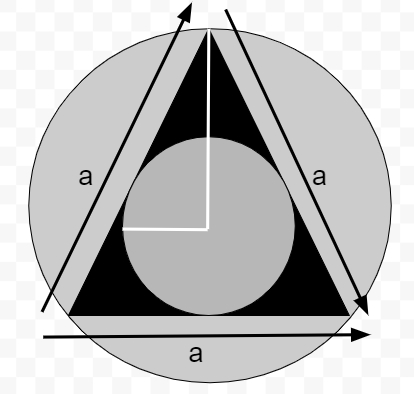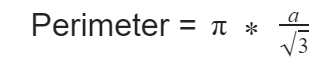# Program to calculate the Area and Perimeter of Incircle of an Equilateral Triangle What is Equilateral Triangle in C?

CServer Side ProgrammingProgramming

## What is Equilateral Triangle?

As the name suggests, equilateral triangle is the one that have equal sides and also it have equal interior angles of 60° each. It is also known as regular triangle because it’s a regular polygon

Properties of equilateral triangle are −

• 3 sides of equal length
• Interior angles of same degree which is 60

## Incircle

Incircle is the circle that lies inside the triangle which means the center of circle is same as of triangle as shown in the figure below. The center of incircle is known as incenter and radius is known as inradius.

Given below is the figure of Incircle of an Equilateral Triangle## Problem

Given with the side of an equilateral triangle the task is to find the area and perimeter of an incircle inside it where area is the space occupied by the shape and volume is the space that a shape can contain.

To calculate area and perimeter of an incircle inside equilateral triangle there is a formula −## Example

Input-: side=6.0
Output-: Area of inscribed circle is :1.046667
Perimeter of inscribed circle is :3.625760

## Algorithm

Start
Step 1 -> define macro as
#define pi 3.14
Step 2 -> Declare function to find area of inscribed circle
float area(float a)
return (a * a * (pi / 12))
step 3 -> Declare function to find Perimeter of inscribed circle
float perimeter(float a)
return (pi * (a / sqrt(3)))
step 4 -> In main()
Declare variable as float a = 6.0
Call area(a)
Call perimeter(a)
Stop

## Example

#include <math.h>
#include <stdio.h>
#define pi 3.14
// function to find area of inscribed circle
float area(float a){
return (a * a * (pi / 12));
}
// function to find Perimeter of inscribed circle
float perimeter(float a){
return (pi * (a / sqrt(3)));
}
int main(){
float a = 6.0;
printf("Area of inscribed circle is :%f\n",area(a));
printf("Perimeter of inscribed circle is :%f",perimeter(a));
return 0;
}

## Output

Area of inscribed circle is :1.046667
Perimeter of inscribed circle is :3.625760